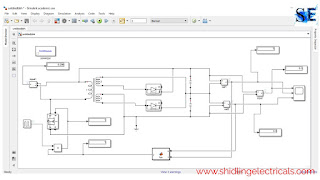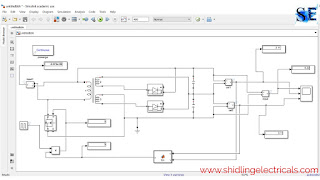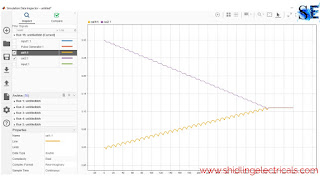# Active Cell Balancing Using A Flyback Converter Simulation In Matlab Simulink

Hello guys, welcome back to my blog. In this article, I will discuss active cell balancing using a flyback converter simulation in Matlab Simulink, I will show simulation, waveforms, Matlab function block code, etc.

Each and everything I will try to explain in a simple way. You can also catch me @ Instagram - Chetan Shidling.

If you need an article on some other topics then comment us below in the comment box. Also, read - Battery management system interview questions and answers.

## Active Cell Balancing Using A Flyback Converter Simulation In Matlab SimulinkAt Initial Stage

The above circuit shows active cell balancing using a flyback transformer. In this technic, I am using capacitors instead of cells and I will make the voltage of each capacitor equal by applying cell balancing technic. I gave the initial voltage of capacitors as 3 and 3.3 as you can see in the above image.

The initial voltage of capacitor 01 is 3 and the initial voltage of capacitor 02 is 3.3, when the cell balancing has applied the voltage across each capacitor will be average of two capacitors, i.e, 3.15 volts.Initial Waveform

As you can see in the above image. The waveform of capacitors voltage. The initial voltage of capacitor 01 and capacitor 02 is 3 and 3.3.

### The active cell balancing circuit consists of;

#### 01. Linear transformer

The input to the linear transformer will be the sum of two capacitor voltage. It is a 1:2 ratio transformer. The secondary side has two winding, each winding gets equal voltage i.e., the average of capacitor voltage.

#### 02. Diodes

In this circuit, the diode conducts only if the anode voltage is greater than the cathode voltage. If the anode voltage is not greater than the cathode voltage than the diode does not conduct.

#### 03. Product

The purpose of the product is to produce a switching pulse to Mosfet when both capacitor voltages are not equal. When the voltage of both capacitors becomes equal than the product gives a low output(0) and hence Mosfet will be turn off and the circuit will be opened.

#### 04. Mosfet

It is used as a switch to make the circuit close or open.

#### 05. Matlab function

In Matlab Function, I wrote the code which will compare the voltage of both capacitors.

#### 06. Current measurement

The current measurement is used to measure the current flowing in the circuit.

#### 07. Voltage measurement

The voltage measurement is used to measure the voltage across the two capacitors, capacitor 01 and capacitor 02.

#### 08. Scope

It is used to display the waveforms of voltage and current.

#### 09. Display

It will display the value of voltage and current as you can see in the above images.

#### 10. Capacitor

Instead of a cell, I am using a capacitor for balancing.

#### 11. Pulse generator

It will produce a pulse to the gate of the Mosfet.

#### Working Of Circuit Diagram

The circuit working is simple, initially, the voltage of capacitor 01 is 3, and the voltage of capacitor 02 is 3.3, to make both capacitor voltage the same we are using active cell balancing. The voltage across the two capacitors is 6.3 which is fed to the linear transformer as input. The secondary side has two windings, both carry equal voltage. When the anode voltage is greater than the cathode then the diode conducts and charges the capacitor. When the anode voltage is less than the cathode then the diode does not conduct and the capacitor only discharges.

The Matlab function will compare the voltage of each capacitor. When the voltage across each capacitor becomes equal the Mosfet turn off and hence circuit will become open.

Now, I will share the circuit diagram and waveform after applying cell balancing.After active cell balancing applied

Now, you can see the voltage on display. The voltage across each capacitor is equal. Inactive cell balancing, the energy of one capacitor is transferred to another capacitor. The 1.5 volts of capacitor 02 is transferred to capacitor 01.The waveform of active cell balancing

The above images show the waveform of active cell balancing. As you can see the voltage becoming equal.